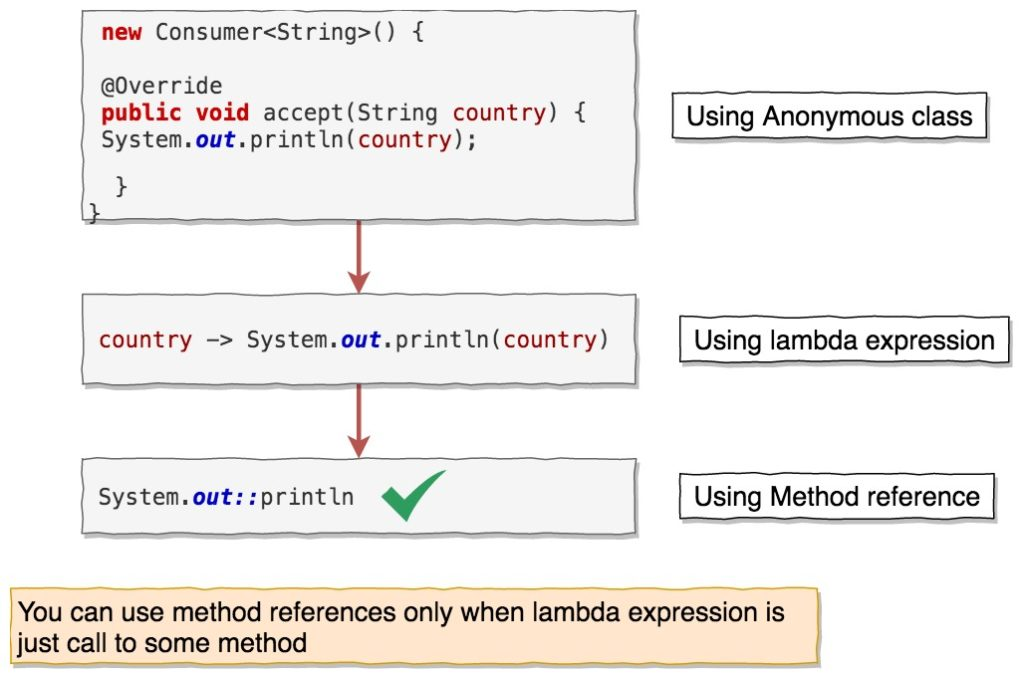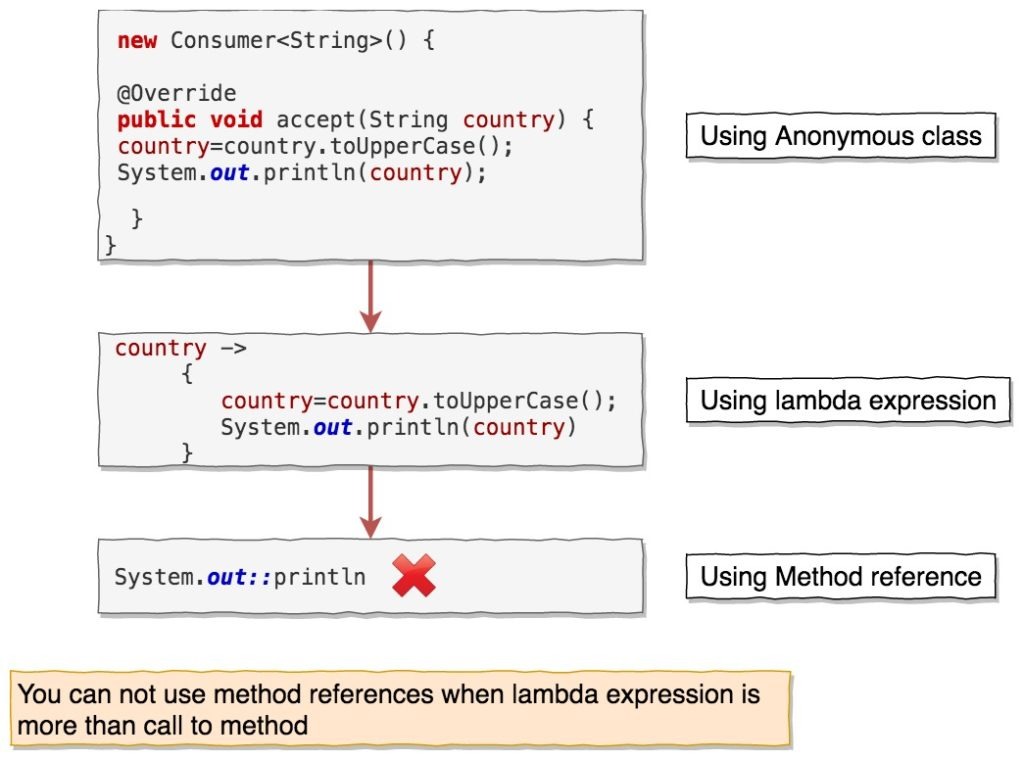# Java 8 Method reference

Java 8 has introduced a lot of new features such as lambda expressions, stream, Method references etc.

In this post, we will see what are Method references and how can we use it. I will try to provide more examples rather than theory.

## Introduction to Method Reference

`Method references` are special types of lambda expressions that execute only one method.
General syntax of method reference:

You might have already guessed that you need to understand lambda expressions first. If you are comfortable with lambda expressions, then let’s move forward.

Let’s understand this with the help of example.
Let’s create class `MethodReferecesLambdaExpressionMain` in which we are going to print list using stream’s foreach method.

Output:

=======================
Using anonymous class
=======================
India
China
Nepal
Russia
=======================
Using lambda expression
=======================
India
China
Nepal
Russia
=======================
Using Method references
=======================
India
China
Nepal
Russia

`stream.foreach()` method takes consumer functional interface as agrument.

Consumer is functional interface that takes a single argument and returns nothing.
We have used consumer functional interface in 3 ways.

1. Using anonymous class
2. Using lambda expression
3. Using method reference

You might already know that you can use lambda expression instead of an anonymous class, but You can use method reference only when the lambda expression just calls to a method.

So if you look at below syntax:

In method reference, we have Class or object before `::` and method name after `::` without arguments.

Did you notice the method reference does not have arguments?
Yes, we don’t need to pass arguments to method reference, arguments are passed automatically internally based on type of method reference.

The below diagrams will make it clearer.
You can use method reference as below:Let’s say you want to convert `country` to `uppercase` before printing it. You can achieve it using `anonymous class` and `lambda expression` but not with method reference.

You can not use method reference as below:You can obviously use the stream’s map() method to convert the `country` to `uppercase` before printing it. I just want to demonstrate when method reference can’t be used.

## Types of method references

There are four types of method references.

1. Reference to static method
2. Reference to instance method of object type
3. Reference to instance method of existing object
4. Reference constructor

### Reference to static method

When you have lambda expression which calls to static method, then you can method reference to static method.

Lambda expression syntax
`(args) -> ClassName.someStaticMethod(args)`
can be converted to
`ClassName::someStaticMethod`

Let’s see this with the help of example.

Create a class name `PowerFunctions`

Function is functional interface that takes a single input T and returns a single output R.

We can call `calculatePowOf2ForList()` as below:

When you run above program, you will get below output:

[1, 4, 9, 16, 25]
[1, 4, 9, 16, 25]
[1, 4, 9, 16, 25]

If you notice,`Function&lt;Integer,Integer&gt; function2 = (num) -&gt; PowerFunctions.power(num);` is of type `(args) -> className.someStaticMethod(args)`
Here,

• `PowerFunctions` is className
• someStaticMethod is `power` method
• `num` is power method argument.

We are calling a static method `power` of class `PowerFunctions` in lambda expression, that’s why we can use it as method reference.

`Function<Integer,Integer> function2 = (num) -> PowerFunctions.power(num);`
we can use
`Function<Integer,Integer> function3 = PowerFunctions::power;`

Here,

• First type parameter of `Function`(Integer) is first parameter of static method `power()`.
• Second type parameter of `Function`(Integer) is return type of static method `power()`.

### Reference to instance method of object type

When you have lambda expression where instance of object is passed and calls to an instance method with/without parameters, then you can use method reference to an instance method with object type.

Lambda expression syntax
`(obj,args) -> obj.someInstanceMethod(args)`
can be converted to
`objectType::someInstanceMethod`

Let’s see this with the help of example.

`BiFunction` is functional interface that takes two arguments and returns single output.

If you notice,`BiFunction<String,Integer,String> bf2 = (t,u) -> t.substring(u);` is of type `(obj,args) -> obj.someInstanceMethod(args)`

Here

• `obj` is of type String.
• someInstanceMethod is String’s `substring()` method.
• args is `beginIndex` for `substring()` method argument.

So `BiFunction<String,Integer,String> bf2 =  (t,u) -> t.substring(u);` can be converted to
`  BiFunction<String,Integer,String> bf3 = String::substring;`

Here,

• First `BiFunction` parameter type(String) is String object itself.
• Second `BiFunction` parameter type(Integer) is argument to `substring()` method
• Third `BiFunction` parameter type(String) is return type of `substring()` method

### Reference to instance method of existing object

When you have lambda expression where instance of object is used to call an instance method with/without parameters, then you can use method reference to an instance method with an existing object.
Lambda expression syntax
`(args) -> obj.someInstanceMethod(args)`
can be converted to
`objectType::someInstanceMethod`

Here `obj` is defined somewhere else and is not part of argument to lambda expression.

Let’s understand with the help of example.

Create a class named `Country.java`.

Create another class `MethodReferenceExistingObjectMain.java`

Output:

[ name = India population = 20000 ]
[ name = India population = 30000 ]
[ name = India population = 40000 ]

Consumer is functional interface which takes single argument and returns nothing.
If you notice, `Consumer<Long> popCons2 =  (population) -&gt; c.setPopulation(population);` is of type `(args) -> obj.someInstanceMethod(args)`

Here

• obj is of type Country and declared somewhere else.
• someInstanceMethod is Country’s setPopulation method.
• args is `population` for setPopulation method argument.

So `Consumer&lt;Long&gt; popCons2= (population) -&gt; c.setPopulation(population);` can be converted to `Consumer&lt;Long&gt; popCons3 = c::setPopulation;`

Here,

• First Consumer parameter type(Long) is argument to setPopulation method.

### Reference constructor

When lambda expression is used to create new object with/without parameters, then you can use reference method constructor.
Lambda expression syntax
`(args) -> new ClassName(args)`
can be converted to
`ClassName::new`

Let’s see with the help of example.
Here we will convert the list to set using method reference.

`Function<List<String>,Set<String>>` is functional interface which will take a list an argument and will return set by calling HashSet constructor `public HashSet(Collection<? extends E> c)`

Output:

[Sneha, Andy, Rohan]
[Sneha, Andy, Rohan]
[Sneha, Andy, Rohan]

If you notice, `Function&lt;List&lt;String&gt;,Set&lt;String&gt;&gt; f2 = (nameList) -&gt; new HashSet&lt;&gt;(nameList);` is of type `(args) -> new ClassName(args)`

Here

• args is of type `list`
• ClassName is `HashSet`

So `Function&lt;List&lt;String&gt;,Set&lt;String&gt;&gt; f2 = (nameList) -&gt; new HashSet&lt;&gt;(nameList);` can be converted to `Function&lt;List&lt;String&gt;,Set&lt;String&gt;&gt; f3= HashSet::new;`

Here,

• First `Function` parameter type(List) is argument to HashSet constructor.

## Excercise

Let’s practice few exercises based on Method reference.

### Excercise:1

Given a list of Integer, you need to find square root of each number in the list and return it as List<Double>.
You need to call Math’s `sqrt` static method to find square root of number and use it as `method reference`.

### Excercise:2

Given a list of String, you need to convert all the String to `uppercase` and then return a new `list`.

### Excercise:3

Given a list of Color objects, you need to sort them of color’s name and return a sorted list of color names(`List<String>`)
Here is the definition of Color class.

## Conclusion

Method references are special type of lambda expression that simply calls a method. It makes code more readable and concise, but if method references lead to any confusion, then there is no point in using them.

That’s all about method references in java.

#### One Response

1.Sachin July 7, 2020